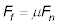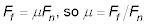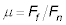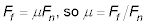##### Physics I Workbook For Dummies with Online PracticeIn the real world, when things slide down ramps, friction is involved, and the force of friction opposes the motion down the ramp. The force of friction is proportional to the force from the ramp that balances the component of gravity that is perpendicular to the ramp. (If this force were not present, the object would sink into the ramp.)

The force that balances the component of gravity that is perpendicular to a surface is called the normal force because its direction is perpendicular to the ramp’s surface. The constant of proportionality is something you have to measure yourself; if the ramp is made of steel, there’s a different amount of friction than if it’s made of sandpaper.

In the equation relating the normal force to the force of friction, Ff, the constant is called the coefficient of friction.## Sample question

1. You’re pushing a refrigerator along your kitchen floor and need to apply 100 degreesN to get it moving. If your refrigerator has a mass of 100 kg, what is the coefficient of friction?

1. The force due to friction is2. 2.The force due to friction, Ff, is 100 N, and the normal force, Fn, is (100)g = 980 N.

3. 3.Use the equationto get the coefficient of friction (note that mu has no units): 100 / 980 = 0.1.

## Practice questions

1. You’re pushing a 15-kg box of books across the carpet and need to apply 100 N. What is the coefficient of friction?

2. You’re pushing a 70-kg easy chair from one room to the next. If you need to apply 200 N, what is the coefficient of friction?

Following are answers to the practice questions:

1. 0.7

1. Solve for mu. The force due to friction is2. The force due to friction, Ff, is 100 N, and the normal force, Fn, is 15g = 148 N.

3. Use the equationto get the coefficient of friction (note that mu has no units): 100/148 = 0.68. Round to 0.7 to account for significant figures.

2. 0.3

1. Solve for mu. The force due to friction is2. The force due to friction, Ff, is 200 N, and the normal force, Fn, is 70g = 690 N.

3. Use the equationto get the coefficient of friction: 200/690 = 0.29. Round to 0.3 to account for significant figures.